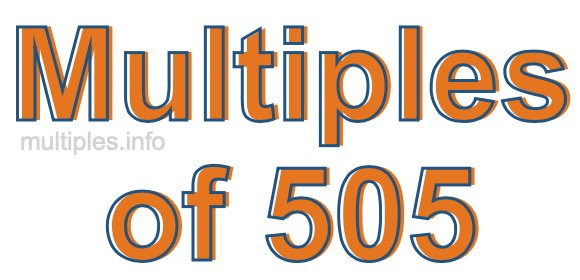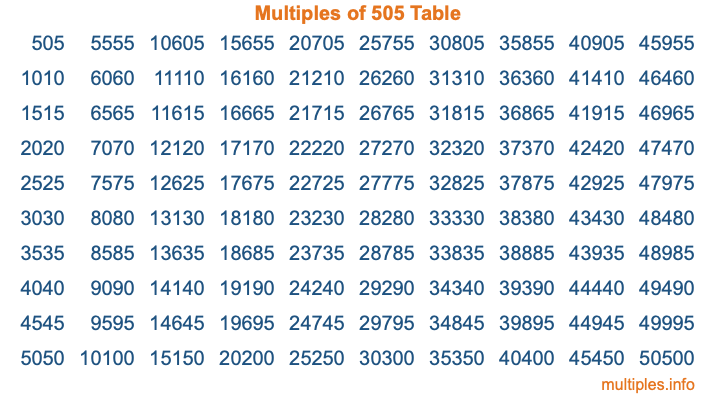Multiples of 505Welcome to the Multiples of 505 page. Here we will first teach you everything you will ever need to know about the multiples of 505, and then give you a study guide summary of everything we taught you to make sure you remember it all. Use this page to look up facts and learn information about the multiples of 505. This page will make you a multiples of five hundred five expert!

Definition of Multiples of 505
Multiples of 505 are all the numbers that when divided by 505 equal an integer. Each of the multiples of 505 are called a multiple. A multiple of 505 is created by multiplying 505 by an integer.

Therefore, to create a list of multiples of 505, you start with 1 multiplied by 505, then 2 multiplied by 505, then 3 multiplied by 505, and so on for as long as you want. Thus, the list of the first five multiples of 505 is 505, 1010, 1515, 2020, and 2525. To see a larger list of multiples of 505, see the printable image of Multiples of 505 further down on this page. We also have a category where you can choose any nth multiple of 505.

Multiples of 505 Checker
The Multiples of 505 Checker below checks to see if any number of your choice is a multiple of 505. In other words, it checks to see if there is any number (integer) that when multiplied by 505 will equal your number. To do that, we divide your number by 505. If the the quotient is an integer, then your number is a multiple of 505.

Is  a multiple of 505?

Least Common Multiple of 505 and ...
A Least Common Multiple (LCM) is the lowest multiple that two or more numbers have in common. This is also called the smallest common multiple or lowest common multiple and is useful to know when you are adding our subtracting fractions. Enter one or more numbers below (505 is already entered) to find the LCM.

Check out our LCM Calculator if you need more details about the Least Common Multiple or if you need the LCM for different numbers for adding and subtraction fractions.

nth Multiple of 505
As we stated above, 505 is the first multiple of 505, 1010 is the second multiple of 505, 1515 is the third multiple of 505, and so on. Enter a number below to find the nth multiple of 505.

th multiple of 505

Multiples of 505 vs Factors of 505
505 is a multiple of 505 and a factor of 505, but that is where the similarities end. All postive multiples of 505 are 505 or greater than 505. All positive factors of 505 are 505 or less than 505.

Below is the beginning list of multiples of 505 and the factors of 505 so you can compare:

Multiples of 505: 505, 1010, 1515, 2020, 2525, etc.

Factors of 505: 1, 5, 101, 505

As you can see, the multiples of 505 are all the numbers that you can divide by 505 to get a whole number. The factors of 505, on the other hand, are all the whole numbers that you can multiply by another whole number to get 505.

It's also interesting to note that if a number (x) is a factor of 505, then 505 will also be a multiple of that number (x).

Multiples of 505 vs Divisors of 505
The divisors of 505 are all the integers that 505 can be divided by evenly. Below is a list of the divisors of 505.

Divisors of 505: 1, 5, 101, 505

The interesting thing to note here is that if you take any multiple of 505 and divide it by a divisor of 505, you will see that the quotient is an integer.

Multiples of 505 Table
Below is an image of the first 100 multiples of 505 in a table. The table is in chronological order, column by column. The first column has the first ten multiples of 505, the second column has the next ten multiples of 505, and so on.The Multiples of 505 Table is also referred to as the 505 Times Table or Times Table of 505. You are welcome to print out our table for your studies.

Negative Multiples of 505
Although not often discussed or needed in math, it is worth mentioning that you can make a list of negative multiples of 505 by multiplying 505 by -1, then by -2, then by -3, and so on, to get the following list of negative multiples of 505:

-505, -1010, -1515, -2020, -2525, etc.

Multiples of 505 Summary
Below is a summary of important Multiples of 505 facts that we have discussed on this page. To retain the knowledge on this page, we recommend that you read through the summary and explain to yourself or a study partner why they hold true.

There are an infinite number of multiples of 505.

A multiple of 505 divided by 505 will equal a whole number.

505 divided by a factor of 505 equals a divisor of 505.

The nth multiple of 505 is n times 505.

The largest factor of 505 is equal to the first positive multiple of 505.

505 is a multiple of every factor of 505.

505 is a multiple of 505.

A multiple of 505 divided by a divisor of 505 equals an integer.

505 divided by a divisor of 505 equals a factor of 505.

Any integer times 505 will equal a multiple of 505.

Multiples of a Number
Here you can get the multiples of another number, all with the same attention to detail as we did for multiples of 505 on this page.

Multiples of
Multiples of 506
Did you find our page about multiples of five hundred five educational? Do you want more knowledge? Check out the multiples of the next number on our list!